# # 关于类型

## # 类型种类

JS 中分为七种内置类型，七种内置类型又分为两大类型：基本类型和引用类型

### # 基本类型

#### # 特点

• 基础类型将内容直接存储在栈中（大小固定位置连续的存储空间）
• 记录的是该数据类型的值，即直接访问
• 保存与复制的是值本身
• 方法中定义的变量都是放在栈内存中
• 使用typeof检测数据的类型 ref 类型判断
``````a = 2;
``````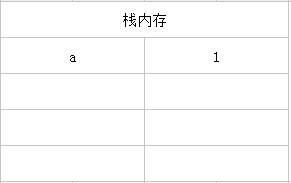### # 引用类型

#### # 特点

``````var a = new Object();
``````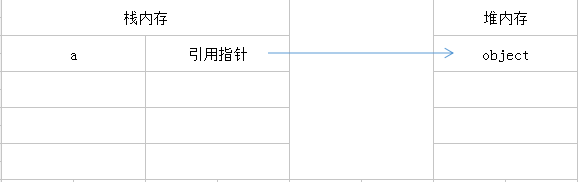• 保存与复制的是指向对象的一个指针
• 使用instanceof检测数据类型 ref 类型判断
• 使用new()方法构造出的对象是引用型 ref new

## # 本地对象、内置对象和宿主对象

### # 本地对象

ECMA-262把本地对象（native object）定义为：独立于宿主环境的ECMAScript实现的对象。

• Object
• Function
• Array
• String
• Number
• Date
• RegExp
• Boolean
• Error
• EvalError
• RangeError
• ReferenceError
• SyntaxError
• TypeError
• URIError

### # 内置对象

ECMA-262把内置对象定义为：由ECMAScript提供实现的、独立于宿主环境的所有对象，在ECMAScript程序开始执行时出现。

ECMA-262定义的内置对象只有两个：Global和Math。（本地对象和内置对象都是独立于宿主对象，根据定义可以看出来内置对象也是本地对象，在JS中所有的内置对象都是本地对象）。

Math对象是我们经常用到的，但是Global就比较少见了。其实我们经常用到Global对象，只是没有用Global这个名字。

Global对象是一个比较特殊的对象，它是一个全局对象，在程序中只有一个，它的存在伴随着整个程序的生命周期，全局对象不能通过名字来访问，但是它有一个window属性，这个属性指向它本身。

## # 类型转换

### # 定义

#### # 静态类型

• 编译时就能发现类型不匹配的错误
• 编译器还可以针对这些信息对程序进行一些优化工作，提高程序执行速度

• 让程序员的精力从思考业务逻辑上分散开来

### # 动态类型

• 编写的代码数量更少
• 给实际编码带来了很大的灵活性

### # 强制类型转换

#### # 转换为字符串

Object.prototype也定义了toString方法，使得所有对象都拥有转换为字符串的能力

``````var n = 1;
n.toString();   // '1'
``````

``````var toString = Object.prototype.toString;
toString.call(new Date); // [object Date]
toString.call(new String); // [object String]
toString.call(Math); // [object Math]
toString.call(undefined); // [object Undefined]
toString.call(null); // [object Null]
toString.call(new MyClass);   // [object Object]
``````

#### # 转换为数字

``````var iNum1 = parseInt("12345red");   //返回 12345
var iNum1 = parseInt("0xA");    //返回 10
var iNum1 = parseInt("56.9");   //返回 56
var iNum1 = parseInt("red");    //返回 NaN
var fNum4 = parseFloat("11.22.33"); //返回 11.22

``````

NaN是JavaScript中唯一一个不等于自己的值。(NaN == NaN) === false

### # 隐形的强制转换

``````const a = '1';
const b = 1;
const c = [1, 2];
const d = [3, 4];
a + 1 // "11"
b + 1 // 2
b + '' // "1"
c + d // "1,23,4"
``````

c 和 d 分别会使用 toString 转为 '1, 2' 与 '3, 4'

``````[] + {} // "[object Object]"
{} + [] // 0
console.log({} + []) //[object Object]
``````
• ==[] + {}== 中，[] 先执行valueOf，返回[],然后再执行toString,返回"", 而 {} 会转为字串 "[object Object]"。
• =={} + []== 中，{} 被当成空区块而无作用，+[] 被当成强制转型为数字 Number([]) （由于阵列是物件，中间会先使用 toString 转成字空串，导致变成 Number('')）而得到 0。
• 但是为何console.log({} + []) //[object Object]和{}+[] 答案不一样就不知道（需要解决）

js——类型转换原理

### # 转换规则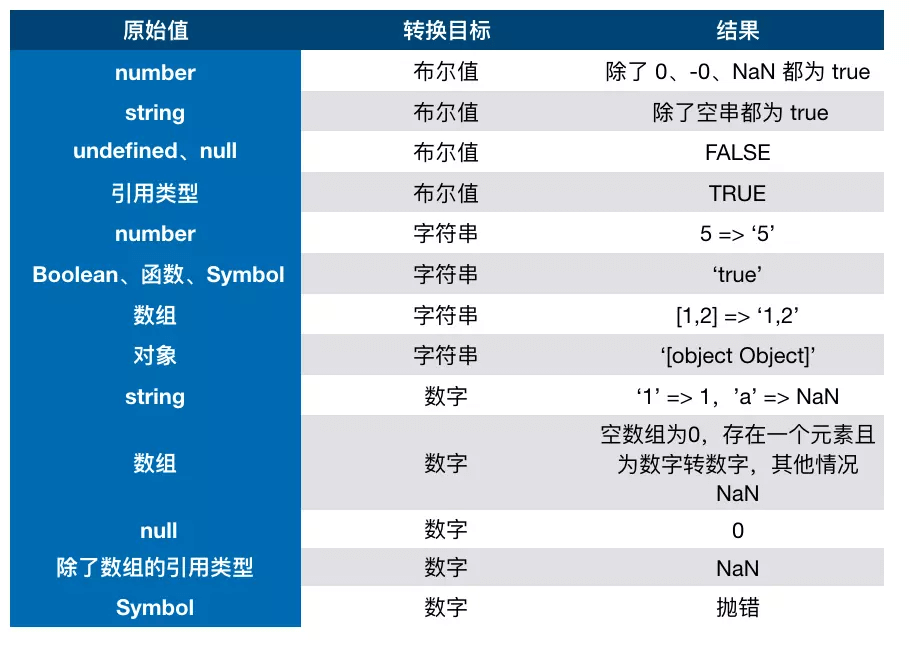## # 类型判断

### # typeof

#### # 概念

Undefined “undefined”
Null “object”

Symbol (ECMAScript 6 新增) “symbol”

isNaN “object”

#### # 题目问题

``````var y = 1, x = y = typeof x;
x;
``````

``````(function f(f){
return typeof f();
})(function(){ return 1; });
``````

`````` var foo = {
bar: function() { return this.baz; },
baz: 1
};
(function(){
return typeof arguments();
})(foo.bar);
``````

`````` var foo = {
bar: function(){ return this.baz; },
baz: 1
}
typeof (f = foo.bar)();
``````

``````var f = (function f(){ return "1"; }, function g(){ return 2; })();
typeof f;
``````

``````  var x = 1;
if (function f(){}) {
x += typeof f;
}
x;
``````

`````` (function(foo){
return typeof foo.bar;
})({ foo: { bar: 1 } });
``````

#### # 题目答案

``````var y = 1, x = y = typeof x;
x;//"undefined"
``````

``````(function f(f){
return typeof f();//"number"
})(function(){ return 1; });
``````

``````  var foo = {
bar: function() { return this.baz; },
baz: 1
};
(function(){
return typeof arguments();//"undefined"
})(foo.bar);
``````

`````` var foo = {
bar: function(){ return this.baz; },
baz: 1
}
typeof (f = foo.bar)();//undefined
``````

``````var f = (function f(){ return "1"; }, function g(){ return 2; })();
typeof f;//"number"
``````

``````var f = (function f(){ return "1"; }, function g(){ return 2; })();
typeof f;//"number"
``````

``````var a = (1,2,3);
document.write(a);//3,会以最后一个为准
``````

``````  var x = 1;
if (function f(){}) {
x += typeof f;
}
x;//"1undefined"
``````

``````(function(foo){
return typeof foo.bar;
})({ foo: { bar: 1 } });
``````

### # instanceof

#### # 定义

instanceof 检测左侧的 proto 原型链上，是否存在右侧的 prototype 原型

``````function _instanceof(A, B) {
var O = B.prototype;// 取B的显示原型
A = A.__proto__;// 取A的隐式原型
while (true) {
//Object.prototype.__proto__ === null
if (A === null)
return false;
if (O === A)// 这里重点：当 O 严格等于 A 时，返回 true
return true;
A = A.__proto__;
}
}
``````

#### # 使用

``````Object.prototype.toString.call()
``````

Undefined object Undefined
Null object Null

Symbol (ECMAScript 6 新增) object symbol

Date object Date

RegExp object RegExp

#### # 类型判断函数

``````var type = function (o){
var s = Object.prototype.toString.call(o);
return s.match(/\[object (.*?)\]/).toLowerCase();
};

['Null',
'Undefined',
'Object',
'Array',
'String',
'Number',
'Boolean',
'Function',
'RegExp'
].forEach(function (t) {
type['is' + t] = function (o) {
return type(o) === t.toLowerCase();
};
});

type.isObject({}) // true
type.isNumber(NaN) // true
type.isRegExp(/abc/) // true
``````

## #`==`vs `===`

### # 两者的异同

• 比较双方都是对象时，只有指向同一个对象才会相等(包含==/===)
• ===要求比较双方类型相同并且值相等
• ==在比较双方类型不同的时候通常会进行隐式类型转换

### #`===` 规律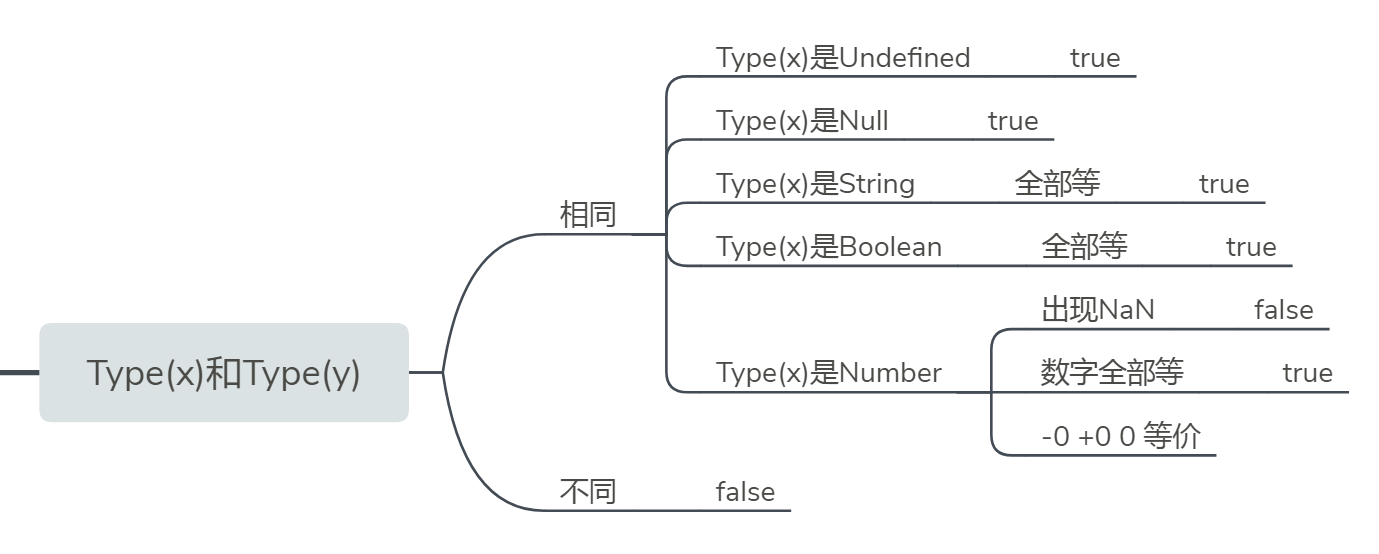• 如果Type(x)和Type(y)不同，返回false
• 如果Type(x)和Type(y)相同
• 如果Type(x)是Undefined，返回true
• 如果Type(x)是Null，返回true
• 如果Type(x)是String，当且仅当x,y字符序列完全相同（长度相同，每个位置上的字符也相同）时返回true，否则返回false
• 如果Type(x)是Boolean，如果x,y都是true或x,y都是false返回true，否则返回false
• 如果Type(x)是Symbol，如果x,y是相同的Symbol值，返回true,否则返回false
• 如果Type(x)是Number类型
• 如果x是NaN，返回false
• 如果y是NaN，返回false
• 如果x的数字值和y相等，返回true
• 如果x是+0，y是-0，返回true
• 如果x是-0，y是+0，返回true
• 其他返回false

### #`==` 规律

• 如果Type(x)和Type(y)相同，返回x===y的结果
• 如果Type(x)和Type(y)不同
• 如果x是null，y是undefined，返回true
• 如果x是undefined，y是null，返回true
• 如果Type(x)是Number，Type(y)是String，返回 x`==`ToNumber(y) 的结果
• 如果Type(x)是String，Type(y)是Number，返回 ToNumber(x)`==`y 的结果
• 如果Type(x)是Boolean，返回 ToNumber(x)`==`y 的结果
• 如果Type(y)是Boolean，返回 x`==`ToNumber(y) 的结果
• 如果Type(x)是String或Number或Symbol中的一种并且Type(y)是Object，返回 x`==`ToPrimitive(y) 的结果
• 如果Type(x)是Object并且Type(y)是String或Number或Symbol中的一种，返回 ToPrimitive(x)`==`y 的结果 其他返回false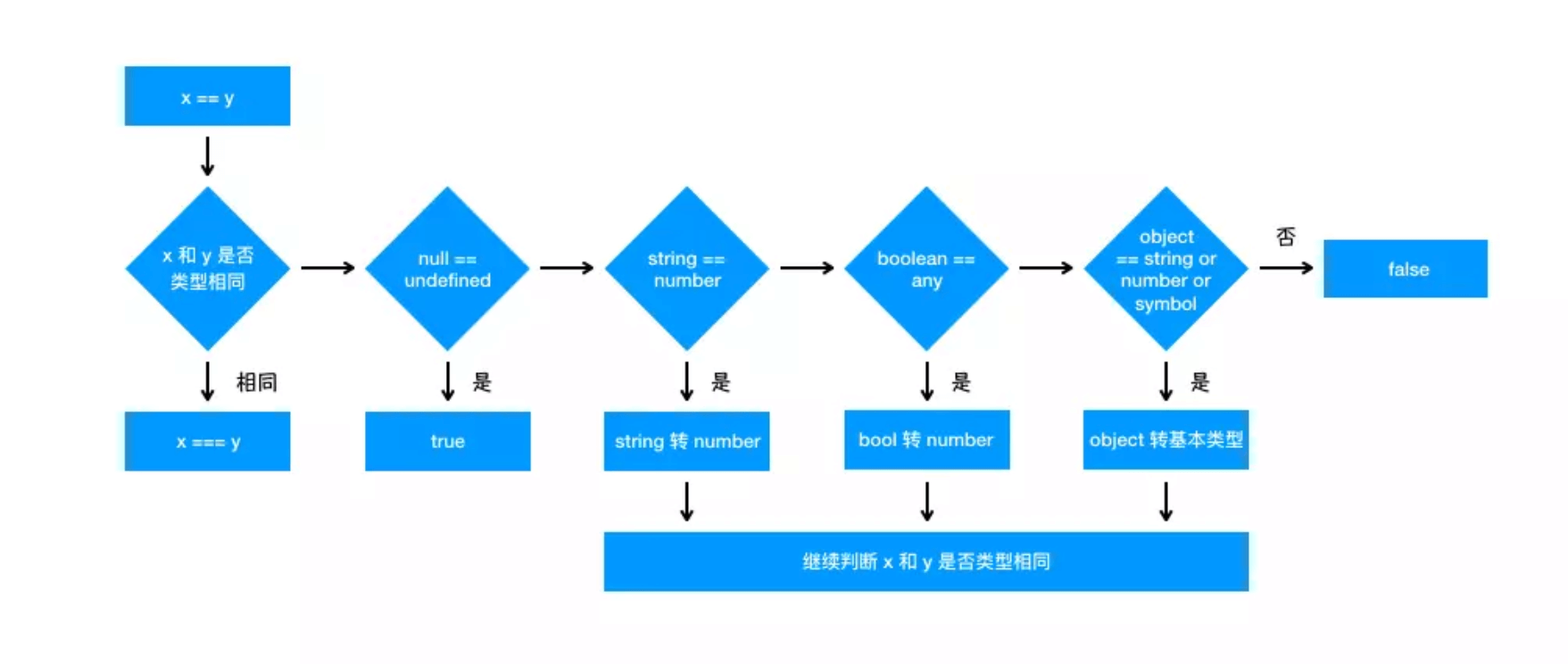## # 浅拷贝与深拷贝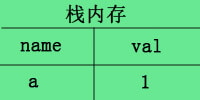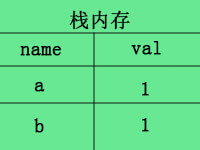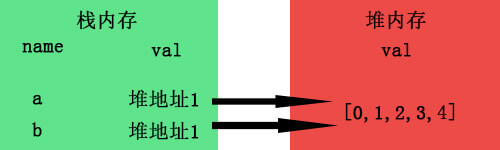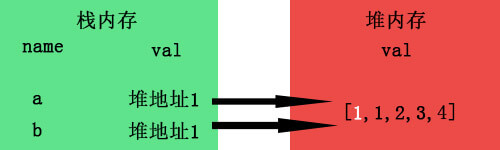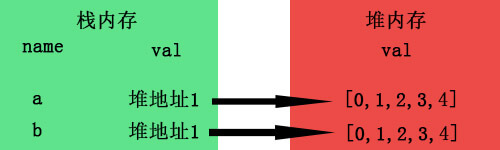### # 浅拷贝的实现方式

#### # 1. 直接赋值一个变量

``````  let obj = {username: 'kobe', age: 39, sex: {option1: '男', option2: '女'}};
let obj1 = obj;
obj1.sex.option1 = '不男不女'; // 修改复制的对象会影响原对象
console.log(obj1, obj);
``````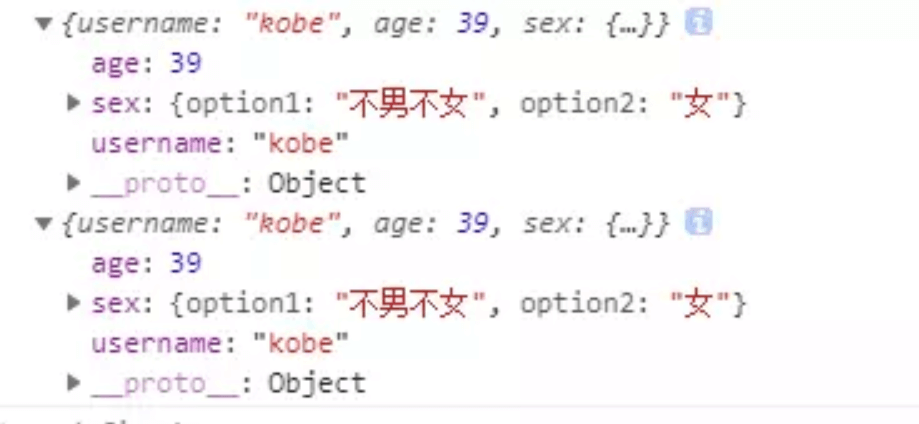#### # 2. Object.assign()

``````let obj = {
};
let obj2 = Object.assign(obj);

``````

#### # 3. Array.prototype.concat()

``````let arr = [1, 3, {
}];
let arr2=arr.concat();
console.log(arr);

``````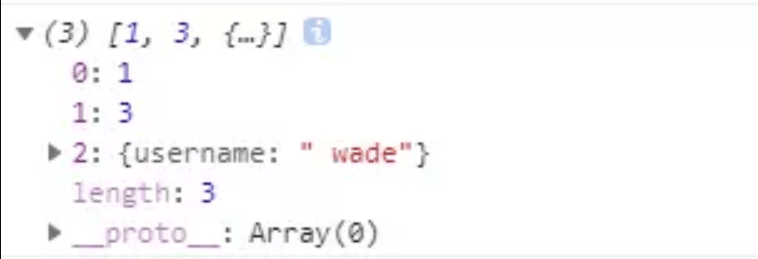#### # 4. Array.prototype.slice()

``````let arr = [1, 3, {
}];
let arr3 = arr.slice();
console.log(arr);

``````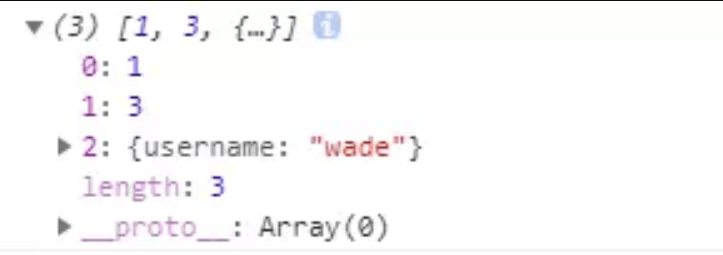• 如果该元素是个对象引用(不是实际的对象)，slice 会拷贝这个对象引用到新的数组里。两个对象引用都引用了同一个对象。如果被引用的对象发生改变，则新的和原来的数组中的这个元素也会发生改变
• 对于字符串、数字及布尔值来说（不是 String、Number 或者 Boolean 对象），slice 会拷贝这些值到新的数组里。在别的数组里修改这些字符串或数字或是布尔值，将不会影响另一个数组。
``````let arr = [1, 3, {
}];
let arr3 = arr.slice();
arr3 = 2
console.log(arr,arr3);

``````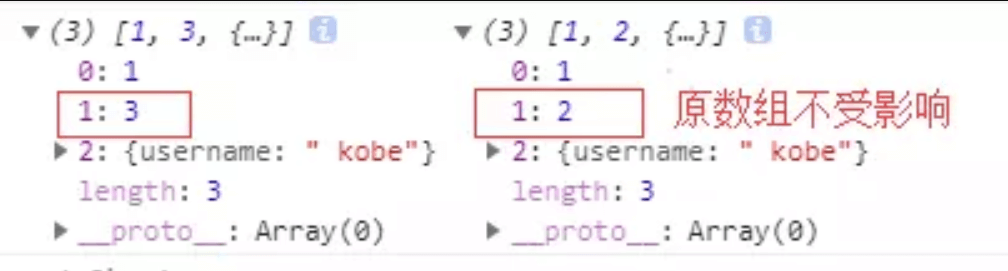### # 深拷贝的实现方式

#### # 1.JSON.parse(JSON.stringify())

``````let arr = [1, 3, {
}];
let arr4 = JSON.parse(JSON.stringify(arr));
console.log(arr, arr4)

``````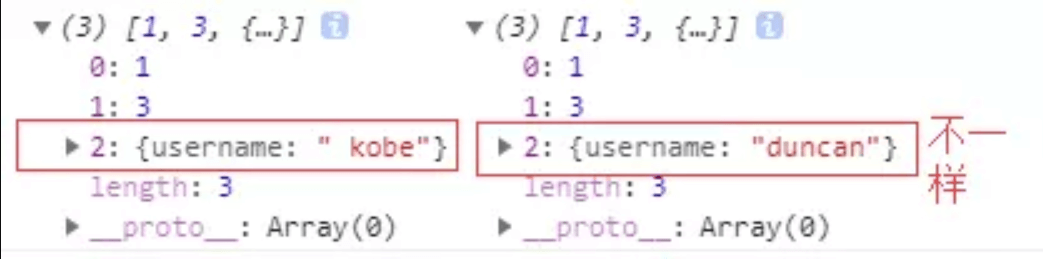``````let arr = [1, 3, {
},function(){}];
let arr4 = JSON.parse(JSON.stringify(arr));
console.log(arr, arr4)

``````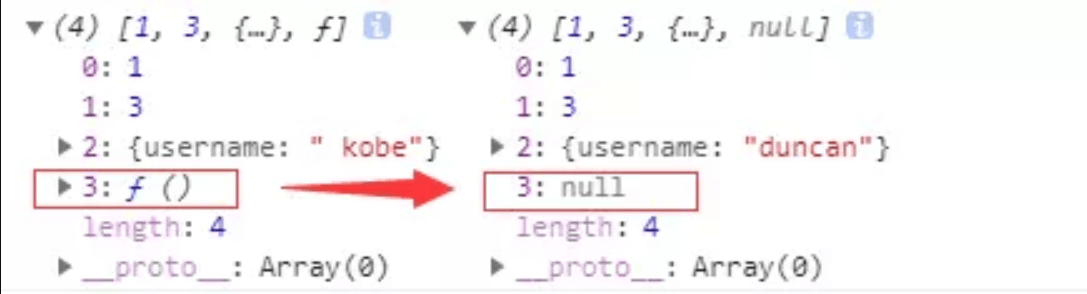#### # 手写递归方法

``````    function extend() {
let name, options, copy, src
let length = arguments.length
let target = arguments || {}
for(let i=1; i<length; i++) {
options = arguments[i]
if(options != null) {
for(name in options) {
copy = options[name]
src = target[name]
if(copy && typeof copy == 'object') {
target[name] = extend(src, copy)
} else if(copy != undefined) {
target[name] = copy
}
}
}
}
return target
}

``````

#### # 深度Object拷贝

``````function extend() {
let name, options, copy, src
let length = arguments.length
let target = arguments || {}
for(let i=1; i<length; i++) {
options = arguments[i]
if(options != null) {
for(name in options) {
copy = options[name]
src = target[name]
if(copy && typeof copy == 'object') {
target[name] = extend(src, copy)
} else if(copy != undefined) {
target[name] = copy
}
}
}
}
return target
}
``````

#### # 3.函数库lodash

``````var _ = require('lodash');
var obj1 = {
a: 1,
b: { f: { g: 1 } },
c: [1, 2, 3]
};
var obj2 = _.cloneDeep(obj1);
console.log(obj1.b.f === obj2.b.f);
// false

``````